## Diffusion Equation

流体流れ, 熱伝導および質量輸送   拡散   拡散方程式

### Fick's Laws

The simplest description of diffusion is given by Fick's laws, which were developed by Adolf Fick in the 19th century:

1. The molar flux due to diffusion is proportional to the concentration gradient.

2. The rate of change of concentration at a point in space is proportional to the second derivative of concentration with space.

#### Fick's First Law of Diffusion

Writing the first law in a modern mathematical form: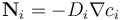where for species i, Ni is the molar flux (mol m-2 s-1), Di is the diffusion coefficient (m2 s-1), and ci is the concentration (mol m-3).

From the continuity equation for mass: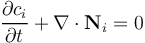we can derive Fick's second law directly: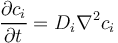This assumes that Di is a constant, which is only true for dilute solutions. This is usually a good assumption for diffusion in solids; diffusion of chemicals in a dilute solution, water, or other typical liquid solvents; and diffusion of dilute (trace) species in the gas phase, such as carbon dioxide in air.

#### Fick's Second Law of Diffusion

Fick's second law of diffusion is a linear equation with the dependent variable being the concentration of the chemical species under consideration. Diffusion of each chemical species occurs independently. These properties make mass transport systems described by Fick's second law easy to simulate numerically.

When modeling diffusion, it is often a good idea to begin with the assumption that all diffusion coefficients are equal and independent of temperature, pressure, etc. This simplification ensures the linearity of the mass transport equations in the modeled domain and often allows simpler correlations to known analytical limits. This assumption can be relaxed once the behavior of a system with all equal diffusion coefficients is well understood.

Dimensional analysis of Fick's second law reveals that, in diffusive processes, there is a fundamental relation between the elapsed time and the square of the length over which diffusion takes place. Understanding this relation is very important for an accurate numerical simulation of diffusion.

### Multi-Component Diffusion

For concentrated solutions or gas mixtures where more than one chemical species is present in significant mass fractions, it is no longer the case that the diffusion coefficient can be treated as constant or composition-independent. The interactivity of the different species' molecules with each other is too prevalent for a physical description to ignore these inter-molecular dependencies. Therefore, the diffusion coefficient becomes a tensor and the equation for diffusion is altered to relate the mass flux of one chemical species to the concentration gradients of all chemical species present. The necessary equations are formulated as the Maxwell-Stefan description of diffusion; they are often applied to describe gas mixtures, such as syngas in a reactor or the mix of oxygen, nitrogen, and water in a fuel cell cathode.

In Maxwell-Stefan diffusion, the sensible choice of dependent variables are not the species concentrations, but rather the species mole or mass fractions (xi and ωi respectively). These are constrained as: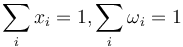and related to the concentration and each other as: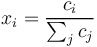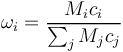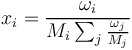where Mi is the relative molar mass (kg mol-1) of species i.

The diffusive mass flux of each species is, in turn, expressed based on the gradients of the mole or mass fractions, using multi-component diffusion coefficients Dik. These are symmetric, so that an n-component system requires n(n-1)/2 independent coefficients to parameterize the rate of diffusion of its components. For four-component or more complex mixtures, these quantities are often unknown. Simplifications can be applied to the Maxwell-Stefan equations in order to employ the equivalent Fick's law diffusivity.

Most often, systems involving concentrated mixtures require convection and momentum conservation (fluid flow) to be solved with diffusion.

Published: January 14, 2015